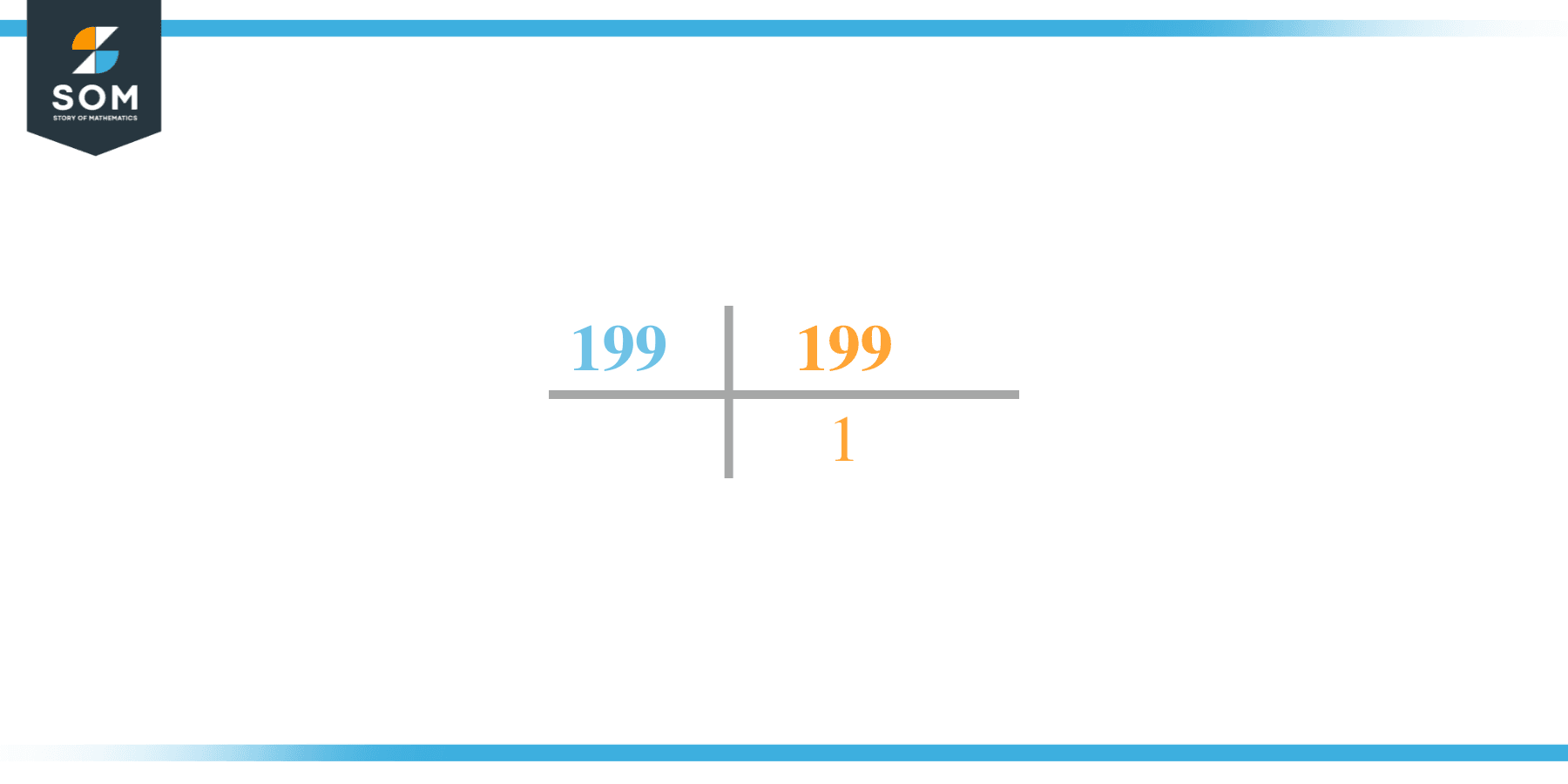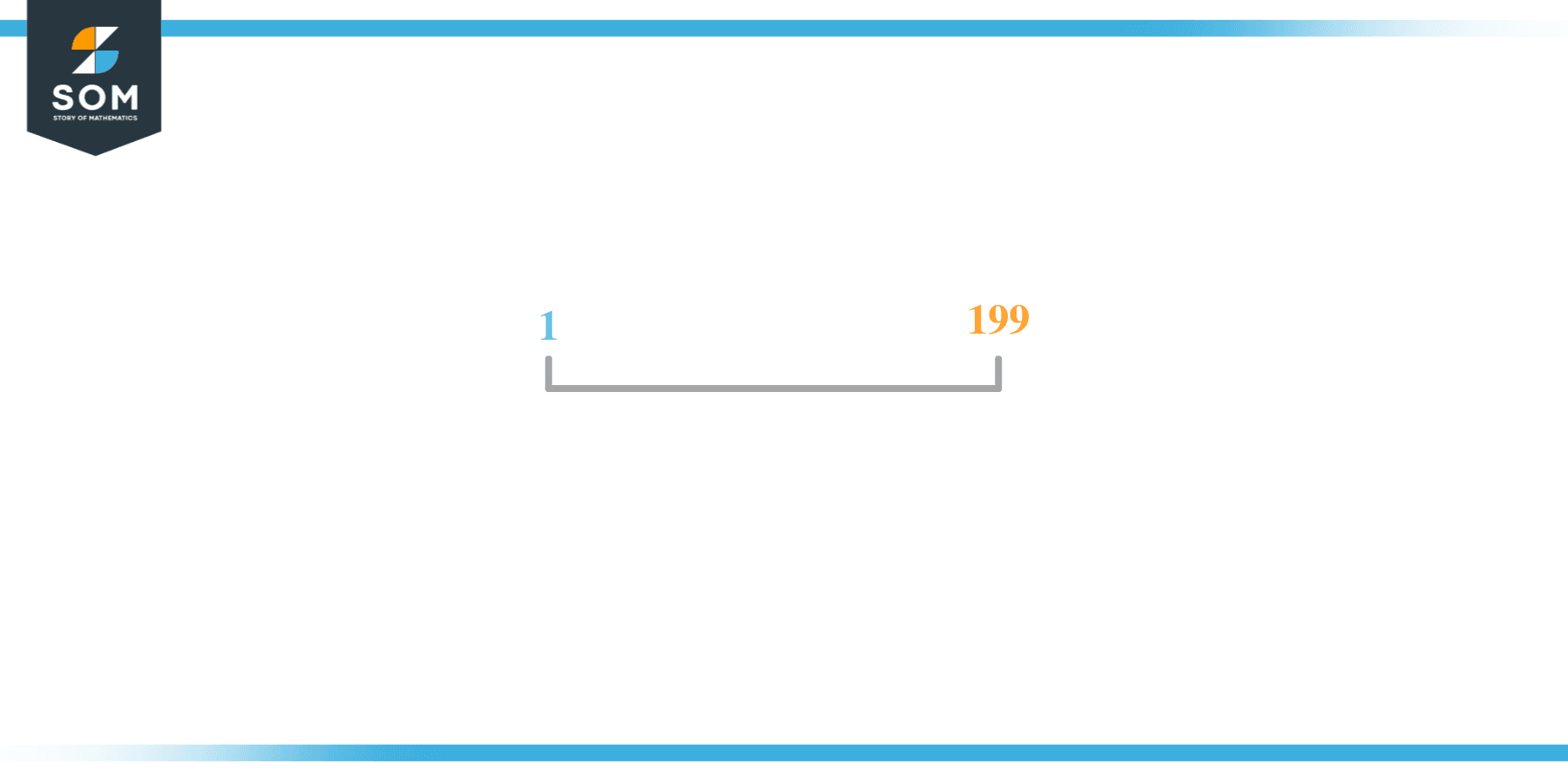# Factors of 199: Prime Factorization, Methods, and Examples

The factors of 199 are the numbers that leave zero as a remainder when 199 is divided from such numbers. Since 199 is a prime number so it only consists of 2 factors. These 2 factors can be both positive as well as negative.Let’s take a look at how to determine these factors.

### Factors of 199

Here are the factors of number 199.

Factors of 199: 1, 199

### Negative Factors of 199

The negative factors of 199 are similar to its positive factors, just with a negative sign.

Negative Factors of 199: -1 and -199

### Prime Factorization of 199

The prime factorization of 199 is the way of expressing its prime factors in the product form.

Prime Factorization: 1 x 199

In this article, we will learn about the factors of 199 and how to find them using various techniques such as upside-down division, prime factorization, and factor tree.

## What Are the Factors of 199?

The factors of 199 are 1 and 199. All of these numbers are the factors as they do not leave any remainder when divided by 199.

The factors of 199 are classified as prime numbers. The prime factors of the number 199 can be determined using the technique of prime factorization.

## How To Find the Factors of 199?

You can find the factors of 199 by using the rules of divisibility. The divisibility rule states that any number, when divided by any other natural number, is said to be divisible by the number if the quotient is the whole number and the resulting remainder is zero.

To find the factors of 199, create a list containing the numbers that are exactly divisible by 199 with zero remainders. One important thing to note is that 1 and 199 are the 199’s factors as every natural number has 1 and the number itself as its factor.

1 is also called the universal factor of every number. The factors of 199 are determined as follows:

$\dfrac{199}{1} = 199$

$\dfrac{199}{199} = 1$

Therefore, 1 and 199 are the factors of 199.

### Total Number of Factors of 199

For 199 there are 2 positive factors and 2 negative ones. So in total, there are 4 factors of 199.

To find the total number of factors of the given number, follow the procedure mentioned below:

1. Find the factorization of the given number.
2. Demonstrate the prime factorization of the number in the form of exponent form.
3. Add 1 to each of the exponents of the prime factor.
4. Now, multiply the resulting exponents together. This obtained product is equivalent to the total number of factors of the given number.

By following this procedure the total number of factors of 199 is given as:

Factorization of 199 is 1 x 199.

The exponent of 1 and 199 is 1.

Adding 1 to each and multiplying them together results in 4.

Therefore, the total number of factors of 199 is 4. 2 are positive and 2 factors are negative.

### Important Notes

Here are some important points that must be considered while finding the factors of any given number:

• The factor of any given number must be a whole number.
• The factors of the number cannot be in the form of decimals or fractions.
• Factors can be positive as well as negative.
• Negative factors are the additive inverse of the positive factors of a given number.
• The factor of a number cannot be greater than that number.
• Every even number has 2 as its prime factor, the smallest prime factor.

## Factors of 199 by Prime FactorizationThe number 199 is a prime number. Prime factorization is a useful technique for finding the number’s prime factors and expressing the number as the product of its prime factors.

Before finding the factors of 199 using prime factorization, let us find out what prime factors are. Prime factors are the factors of any given number that are only divisible by 1 and themselves.

To start the prime factorization of 199, start dividing by its smallest prime factor. First, determine that the given number is either even or odd. If it is an even number, then 2 will be the smallest prime factor.

Continue splitting the quotient obtained until 1 is received as the quotient. The prime factorization of 199 can be expressed as:

199 = 1 x 199

## Factors of 199 in PairsThe factor pairs are the duplet of numbers that when multiplied together result in the factorized number. Factor pairs can be more than one depending on the total number of factors of the given numbers.

For 199, the factor pairs can be found as:

199 = 1 x 199

The possible factor pair of 199 is given as (1, 199).

All these numbers in pairs, when multiplied, give 199 as the product.

The negative factor pairs of 199 are given as:

199 = -1 x -199

It is important to note that in negative factor pairs, the minus sign has been multiplied by the minus sign due to which the resulting product is the original positive number. Therefore, -1 and -199 are called negative factors of 199.

The list of all the factors of 199 including positive as well as negative numbers is given below.

Factor list of 199: 1, -1, 199, and -199

## Factors of 199 Solved Examples

To better understand the concept of factors, let’s solve some examples.

### Example 1

How many factors of 199 are there?

### Solution

The total number of Factors of 199 is 4.

Factors of 199 are 1 and 199.

### Example 2

Find the factors of 199 using prime factorization.

### Solution

The prime factorization of 199 is given as:

199 $\div$ 199 = 1

So the prime factorization of 199 can be written as:

199 = 1 x 199